International Journal of Intelligent Information Systems
Volume 5, Issue 3-1, May 2016, Pages: 23-27

Applying the SAC Algorithm to Extract the Cardiologic Indicators of an Athlete's Level

Mohamed Hamlich1, Mohammed Ramdani2

1Electrical Engineering Department of Hassan II University, ENSAM, Casablanca, Morocco

2Computer Science Lab of Hassan II University, FSTM, Mohammedia, Morocco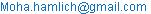(M. Hamlich)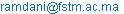(M. Ramdani)

Mohamed Hamlich, Mohammed Ramdani. Applying the SAC Algorithm to Extract the Cardiologic Indicators of an Athlete's Level. International Journal of Intelligent Information Systems. Special Issue: Academic Research for Multidisciplinary. Vol. 5, No. 3-1, 2016, pp. 23-27. doi: 10.11648/j.ijiis.s.2016050301.13

Received: December 19, 2015; Accepted: December 21, 2015; Published: June 18, 2016

Abstract: The objective of this paper is to identify the parameters that determine the level (high or low) of an athlete. The developed method is based on the algorithms of ant colonies. In this paper We will focus on the application of an algorithm named: SAC "Scout Ant for Clustering". This method is an extension of existing data clustering algorithms (ACO) based on ant colonies. The clusters’ separation test was improved by using the probabilities determined in step search of the best path between all instances. The SAC method treated any data sets (heterogeneous attributes: continuous and nominal) and represents each cluster by its prototype. This is determined for each cluster and it is the closest instance to all elements of the cluster. This method will be applied to cardiological data, which are taken on athletes.

Keywords: Ant Colonies, Clustering, Heterogeneous Data, SAC Algorithm, Level Athlete

Contents

1. Introduction

The ant colony algorithms simulate the behavior of real ants using a colony of artificial ants to find the optimal path between the data . The motivation of this work is to develop a method based on ant colonies that can handle heterogeneous data with continuous class.  We developed the algorithm named Fuzzy Ant Miner  that extracts fuzzy rules  from the training data. It processes the data with nominal class  and has the disadvantage of not treating the data as continuous class . We propose a new data classification method based on ant colony algorithms. This method divides the heterogeneous data into homogeneous groups.

A cluster is a collection of objects that are similar to each other and are different objects belonging to other groups.

Our method SAC  is based on ACO algorithm . It is treated in three steps:

Finding the optimal path by ants.

Separation of data into homogeneous groups;

Calculation of the prototype of each group.

The search for the best path is made in the same manner as that used in the ACO method. This step is to compare the paths made by all the ants, crossing all the game data, and select the best in terms of distances.

The originality of our method is that it organizes the data in the form of clusters by using a probabilistic criterion, which depends on the distance and the amount of pheromone deposited by the ant.

Moreover calculating the center of gravity by ACO method assumes that all attributes are continuous, whereas in most of the existing data, attributes are heterogeneous.

Our method will determine a prototype of each cluster; it is the most representative element.

The objective of this study is to have rules that determine the level (beginner or high) of an athlete from cardiological measures taken. The study was performed on 60 athletes from the sport's school (ENS) OF Casablanca. 20 qualified students that beginners just joined the school, 20 qualified students of intermediate level as they passed a year in school and 20 high-qualified students as they passed more than a year in school. The measurements were performed in the cardiology department of the CHU Ibnou Rochd.

The rest of the paper is organized in four sections:

The first is devoted to the explanation of data aggregation principle. In the following, we give the algorithmic structure of our method, the class diagram and graphics interfaces. In the third section, we give the results of our method applied to real data. Then the last section is devoted to classify seventy athletes into three groups according to their sporting level (low, medium or high) according to their cardiological characteristics.

2. Scout Ant for Clustering

A. Finding the best path using the SAC method.

The objective of the first step is to find a good path connecting all points of the data set using the ACO algorithm . This is equivalent to building a graph whose nodes represent all the data analyzed . The probability that k ant, which occupies the i position, moves to the j position, is calculated by the following function :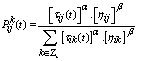(1)

Zk is a list of data not visited by ant k.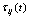is the amount of pheromone in the transition between data i and j.

α is the intensity of the level of pheromone.

β is the data visibility.

ηij is the inverse of the distance between data i and j.

B.  Criteria for separating groups.

After finding the best path by ants in the first stage, the method starts a new phase, which aims to separate the data into homogeneous groups. The objective of this next step is to separate data into homogeneous groups. Clustering  is the act of cutting a set of objects into groups (clusters) so that the characteristics of objects in the same cluster are similar and that the characteristics of objects in different clusters are distinct. We have introduced a new criterion for the separation of homogeneous groups based on the transition probabilities between the data of the optimal path. The method seeks the lowest transition probability among all calculated in the optimal path. The first boundary between the two groups is considered between the given i and j given. Then we redo the same operation in each group so that there is a transition probability ε lower than a value entered by the user: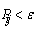(2)

ε is a parameter called attachment coefficient and its range is (0, 1).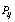is the transition probability (Equation 2) between Examples i and j.

This criterion considers the separation distances and levels of pheromone. The processing time of the separation of groups will be improved compared to ACO algorithms:

the probabilities have been already calculated in the first step.

The criterion of separation does not depend only on the distance but also depends on the amount of pheromone deposited by ants.

The illustration of this separation step is shown in Figure1 where probabilities P16 and P53 are below ε. None of the other probabilities justifies this condition.

C.  Cluster’s prototype.

Once the groups consisting, the method determines a prototype of each cluster based on the distances (Equation 3.3) between each cluster member other data. The prototype of a cluster is the one closest to all cluster data in question. For each element, the method computes the distance separating it from each point in the same cluster, and then calculates the sum of these distances. The prototype will be the element for which this sum is minimal. It will be the representative element of all cluster data.

3. Scout Ant for Clustering Algorithm

The pseudo-code shown in Figure 3.1 shows the various processing steps of our SAC method. The user enters the number of ants, the value of the attachment coefficient and the initial level of pheromone deposited in any position. Default values are available to the user.

Inside the loop (For), each ant finds a path. If the overall length of this path is less than the best path, it will be stored as the new best path. The transition probabilities are stored and pheromones levels will be updated in all positions so that these levels in the nodes visited by the ants will be strengthened and unvisited nodes will be reduced (evaporation of pheromone). Thus, this loop is the best path among all data based on the transition probabilities (Equation 3.1).

The second loop (While) partitions data into homogenous groups on the basis of a new criterion (Equation 3.2). For each group, the method determines the most representative element which is the closest of all cluster.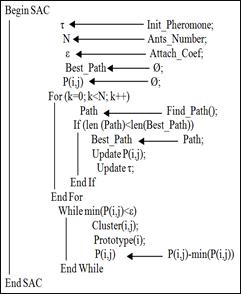Fig. 1. Pseudo code of SAC algotithm.

4. Applications

2.1.UCI datasets

To confirm the results obtained by our method, a comparative study was conducted on real data. In this study, we did tests on standard databases obtained from the data warehouse: UCI. .

The algorithms based on data clustering existing ant colonies treat non heterogeneous datasets. To compare our method with these algorithms, we were forced to experiment on datasets whose attributes are the same type.

We first start by comparing our method with ACOC algorithms "Ant Colony Optimization Clustering Algorithm" and KHM "K- Harmonic Means Clustering algorithm" on datasets " Nursery " and " Solar Flare ". Indices different validation algorithms are grouped: entropy and F- measure.

Then we make comparisons with the ACA algorithms "Ant Clustering Algorithm" and K-means on the data sets "Iris," "Ionosphere", "Pima" and "Wine" using as validation indices: F-measure and SSE (sum of error squares).

A. Comparison of SAC algorithm with ACOC and KHM

For different numbers of ants, we set the attachment coefficient ε to obtain different numbers of clusters. The results obtained show that the SAC method generated partitions that have the lowest average value of entropy and the largest average value of the F-measure factor. Moreover, we note that the best scores of the SAC algorithm are those containing four and five clusters. This can be explained by the fact that the number of clusters approaches the actual number of class data sets used.

"Fig. 2," and "Fig. 3," show, respectively, the average values of validation indices: entropy and F-measure for the Nursery dataset. "Fig. 4," and "Fig. 5," show, respectively, the average values of validation indices: entropy and F-measure for the Solar dataset.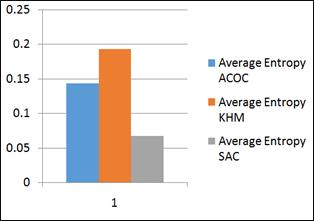Fig. 2. Average values of entropy (Nursery dataset).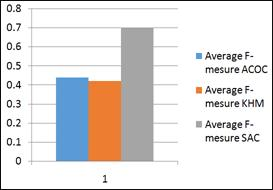Fig. 3. Average values of F-mesure (Nursery dataset).Fig. 4. Average values of entropy (Solar dataset).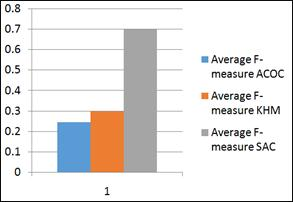Fig. 5. Average values of F-mesure (Solar dataset).

2.2. Comparison of SAC algorithm with k-means and ACA

In these experiments, we measured two-factor validation  of clustering methods:

F-measure: external validation Factor.

SSE: internal validation Factor.

ACA, k-means and SAC algorithms are experienced on four UCI datasets: Iris, Wine, Ionosphere and Pima. The graph below shows the results obtained.Fig. 6. Indices validations.

The results show that the method that presents the lowest average SSE and the highest F-measure is our SAC method.

5. Cardiologic Indicators of an Athlete's Level

A. Dataset.

The data were provided by Abdenasser DRIGHIL, MD: Professor and consultant cardiologist, department of cardiology Ibn Rochd University Hospital. There are two database instances 60 each. The first contains measurements taken before physical effort and the second after physical exertion.

Each database consists of 28 digital and rated class attributes that can have 3 values: d (beginner), m (average level) and h (high level). After a statistical study, and in consultation with the expert, we have qualified the group of average students as belonging to the group of beginners. So, beginners are numbered 0 to 39, and top athletes 40 to 59.

Moreover, and according to a statistical study using SPSS software, we found that only five attributes that change depending on the physical effort and according to the class. So we created a new database that consists of five attributes (MASSEVGPR, SVDPR, SSPR, DHOGPR and TDEPR) and a class (D or H).

B.  Application of the SAC method before physical effort.

After getting the optimal path, the method separated the data into two clusters:

First cluster: beginner.

The prototype of high level athletes is the number 42 whose object attribute values are:

MASSEVGPR=26.85027531.

SVDPR=13.0.

SSPR=7.0.

DHOGPR=35.0.

TDEPR=271.0.

Class=d.

Second cluster: high-level athlete.

The prototype of high level athletes is the number 42 whose object attribute values are:

MASSEVGPR=44.56758309.

SVDPR=16.0.

SSPR=6.0.

DHOGPR=43.0.

TDEPR=146.0.

Class= h.

C.  Application of the SAC method after physical effort.

First cluster: beginner.

The prototype of high-level athletes is whose object attribute values are:

MASSEVGPS=33.70665378.

SVDPS=18.0

SSPS=8.0.

DHOGPS=34.0.

TDEPS=163.0.

Class= d.

Second cluster: high-level athlete.

The prototype of high-level athletes is whose object attribute values are:

MASSEVGPS=45.79442898.

SVDPS=22.0.

SSPS=13.0.

DHOGPS=35.

TDEPS=173.0.

Class=h.

D.  Results interpretation.

We note that the class of the first cluster prototype is beginner. As against the class of the second cluster is high. Those latter shows that the method well separates the two types of athletes.

After seeing the results, the expert has preferred the results obtained by the SAC method as representative prototypes of clusters instead of the results obtained by several classification methods. The only results that coincide with his vision in this database are those of the SAC method.

Conclusion

We proposed a learning method based on ant colonies to treat databases whose class is continuous values. The methods of clustering based ant colony data can only handle the same type of data. Our method takes into account the attributes of continuous type, nominal or ordinal in the data grouping process.

In the group separation process, we used a new probabilistic criterion. This criterion is based on the transition probabilities previously calculated in step search of the best path between all instances. Once determined clusters, the method proceeds to the election of a representative element of each cluster (prototypes). This element is the closest to all instances belonging to the same cluster.

To validate our method, it was tested on artificial data distributed differently. The data distributed in a spherical manner were not properly separated into homogeneous groups. This is a very important feature of our method because of the very popular methods of data clustering do not possess. The results coincide with our expectations in terms of number of clusters and prototypes.

To evaluate our method, it was compared with other algorithms on real data from the UCI repository. The measured value of the index validation f-measure is the largest for the SAC method. It also has the lowest value of entropy.

We applied the method to two real SAC medical databases retrieved the cardiology department at the University Hospital Ibn Rochd. The method has generated for both databases clusters whose prototypes justify the rules obtained by Fuzzy Ant - Miner. A new injection given in the SAC method will be assigned to the cluster that is the closest to him. The cluster is represented by its prototype.

These encouraging results show that our method is effective for different types of databases.

References

1. M.Hamlich,and M.Ramdani, "Data classification by Fuzzy Ant-Miner", IJCSI International Journal of Computer Sciences issues, Vol 9, Issue 2, N° 3, Marsh 2012, ISSN (Online) 1694-08 14.
2. M.Hamlich, and M.Ramdani, «Improved ant colony algorithms for data classification», Complex Systems (ICCS), 2012, Agadir, ISBN: 978-1-4673-4764-8, IEE Xplore.
3. D.Dubois, H.Prade (1996). What are fuzzy rules and how to use them. Fuzzy Sets and Systems, Vol. 84(2), pp. 169-186.
4. Y.Kao and K.Cheng, "An ACO-Based Clustering Algorithm", Tatung University, Taipei, Taiwan (2006).
5. K.Socha, ACO for Continuous and Mixed-Variable Optimization, Proceedings of the Fourth International workshop on Ant Colony Optimization and Swarm Intelligence, Brussels, Belgium, 2004.
6. D.Costa and A.Hertz, "Ants Can Colour Graphs." Journal of the Operational Research Society, 48, 295-305, 1997.
7. M.Divyavani, T.Amudha, "Comparing the Clustering Efficiency of ACO and K-Harmonic Means Techniques", International Journal of Computer Science. Engineering and Applications (IJCSEA) Vol. 1, No. 4, August 2011.
8. M.Hamlich and M.Ramdani, «Applying the Fuzzy Ant-Miner algorithm to extract the success indicators of Balloon Dilation», Congrès International sur les Sciences et Technologies de l’Information et de la Communication (CISTIC2014), Decembre 02-04, 2014.
9. M.Hamlich and M.Ramdani, "Scout Ants for Clustering", Journal of Theoretical and Applied Information Technology (JATIT), September 2013 -- Vol. 55. No. 1--2013.
10. U.Maulik, and S.Bandyopadhyay, (2002) "Performance evaluation of some clustering algorithms and validity indices," IEEE Transactions on Pattern Analysis and Machine Intelligence, vol. 24, no. 12.
11. M.Hamlich and M.Ramdani, "Fuzzy Ant-Miner", IADIS European Conference Data Mining 2012, Lisboa Portugal, ISBN 978-972-8939-69-4.
12. G,A.Chan,and A.Freitas, "A new classification-rule pruning procedure for an ant colony algorithm", Lecture Notes in Artificial Intelligence 2005, 3871 25–36.
13. RUI XU and DONALD C. WUNSCH, II, "Clustering", Published by John Wiley & Sons, Inc., Hoboken, New Jersey, 2009 by Institute of Electrical and Electronics Engineers, Library of Congress Cataloging- in-Publication Data is available. ISBN: 978-0-470-27680-8.

 Contents 1. 2. 3. 4. 5.
Article ToolsAbstractPDF(847K)